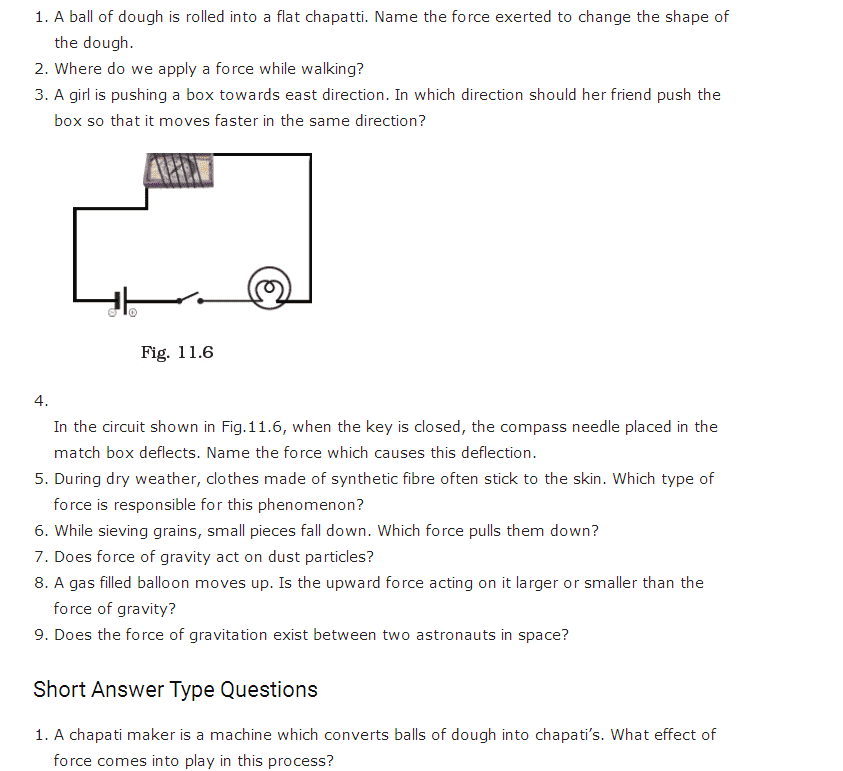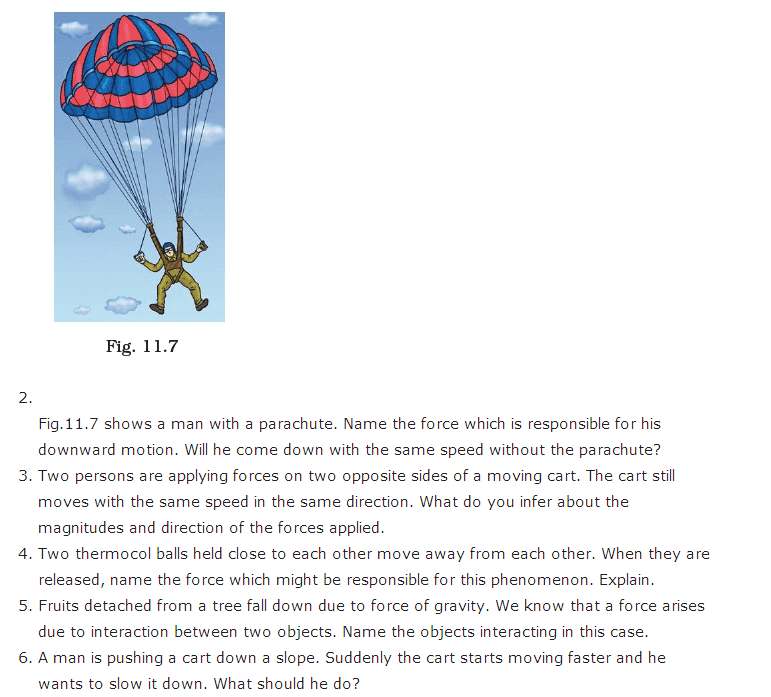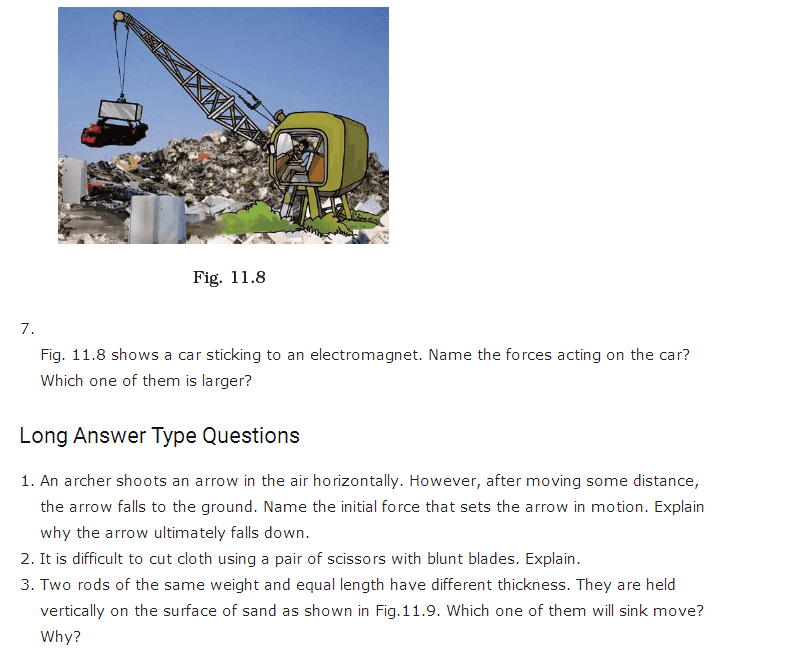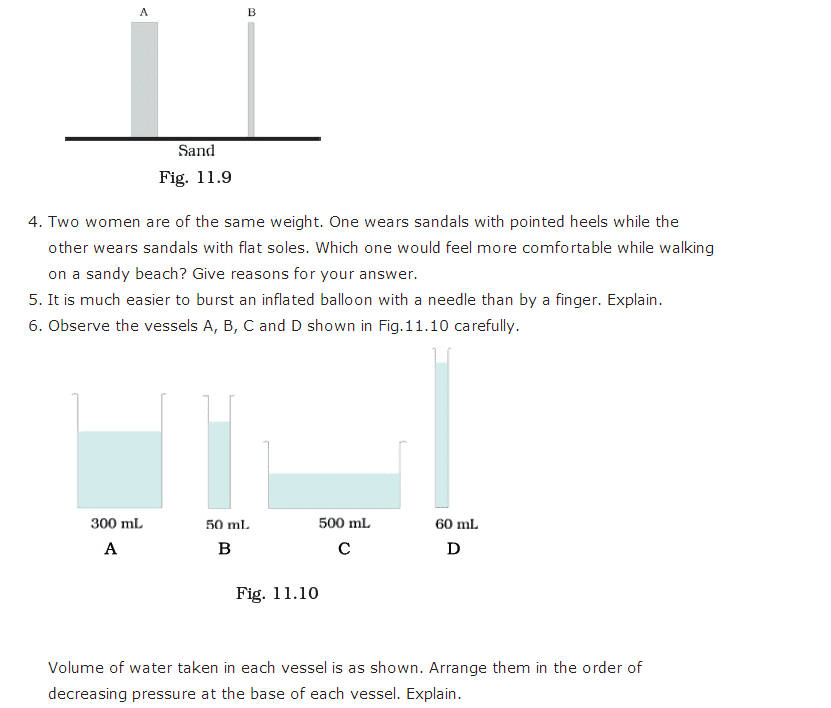# Important Questions for Class 8 Science Chapter 11 - Force and Pressure

Class 8 Science important questions for Chapter 11 – Force and Pressure talks about force, contact force, electrostatic force, friction, gravitational force, pressure, etc. Here we have provided important questions for Class 8 Science Chapter 11 for students so that they don’t waste time making notes of important questions. These important questions are prepared by subject matter experts by referring to Science textbook of Class 8. While preparing for exam, students can refer to these important questions to memorize necessary concepts from this chapter. It guides students to know the topics where they need to concentrate more.

## Important Questions for Class 8 Science Chapter 11 – Force and Pressure PDF### CBSE Class 8 Science Chapter 11 MCQ Type Questions

1. What is the force exerted by the earth to pull the object towards itself known as?
(a) gravitational force
(b) electrostatic force
(c) muscular force
(d) contact force

2. Two objects repel each other. Which among the following is the cause of  this repulsion?
(a) electrostatic force only
(b) frictional force only
(c) magnetic force only
(d) either a magnetic or an electrostatic force

3. What is a push or pull on an object called?
(a) Pressure
(b) Push-pull
(c) Force
(d) All the above